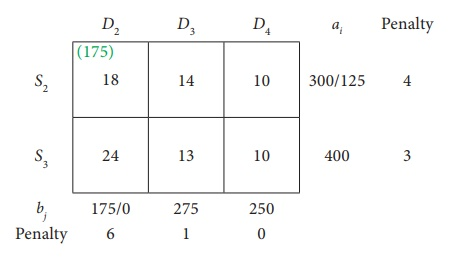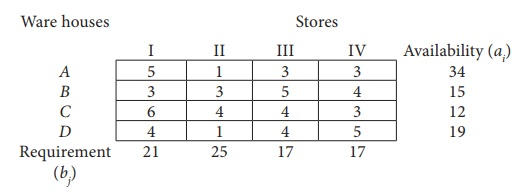Home | | Business Maths 12th Std | Methods of finding initial Basic Feasible Solutions: VogelŌĆÖs Approximation Method(VAM)

# Methods of finding initial Basic Feasible Solutions: VogelŌĆÖs Approximation Method(VAM)

Operations Research: Transportation Problem: Methods of finding initial Basic Feasible Solutions: VogelŌĆÖs Approximation Method(VAM)

## Method: VogelŌĆÖs Approximation Method(VAM)

VogelŌĆÖs approximation method yields an initial basic feasible solution which is very close to the optimum solution.Various steps involved in this method are summarized as under

Step 1: Calculate the penalties for each row and each column. Here penalty means the difference between the two successive least cost in a row and in a column .

Step 2: Select the row or column with the largest penalty.

Step 3: In the selected row or column, allocate the maximum feasible quantity to the cell with the minimum cost.

Step 4: Eliminate the row or column where all the allocations are made.

Step 5: Write the reduced transportation table and repeat the steps 1 to 4.

Step 6: Repeat the procedure until all the allocations are made.

Example 10.5

Find the initial basic feasible solution for the following transportation problem by VAMSolution:

Here Ōłæai = Ōłæbj = 950

(i.e) Total Availability =Total Requirement

Ōł┤The given problem is balanced transportation problem.

Hence there exists a feasible solution to the given problem.

First let us find the difference (penalty) between the first two smallest costs in each row and column and write them in brackets against the respective rows and columnsChoose the largest difference. Here the difference is 5 which corresponds to column D1 and D2. Choose either D1 or D2 arbitrarily. Here we take the column D1 . In this column choose the least cost. Here the least cost corresponds to (S1, D1) . Allocate min (250, 200) = 200units to this Cell.

The reduced transportation table isChoose the largest difference. Here the difference is 5 which corresponds to column D2. In this column choose the least cost. Here the least cost corresponds to (S1, D2) . Allocate min(50,175) = 50 units to this Cell.

The reduced transportation table isChoose the largest difference. Here the difference is 6 which corresponds to column D2. In this column choose the least cost. Here the least cost corresponds to (S2, D2) .

Allocate min(300,175) = 175 units to this cell.

The reduced transportation table isChoose the largest difference. Here the difference is 4 corresponds to row S2. In this row choose the least cost. Here the least cost corresponds to (S2, D4) . Allocate min(125,250) = 125 units to this Cell.

The reduced transportation table isThe Allocation isThus we have the following allocations:Transportation schedule :

S1ŌåÆ D1, S1ŌåÆD2, S2ŌåÆD2, S2ŌåÆD4, S3ŌåÆD3, S3ŌåÆD4

This initial transportation cost

= (200 ├Ś 11) + (50 ├Ś13) + (175 ├Ś 18) + (125 ├Ś10) + (275 ├Ś13) + (125 ├Ś10)

=  Ōé╣ 12,075

Example 10.5

Obtain an initial basic feasible solution to the following transportation problem using VogelŌĆÖs approximation method.Solution:

Here Ōłæai = Ōłæbj = 80 (i.e) Total Availability =Total Requirement

Ōł┤The given problem is balanced transportation problem.

Hence there exists a feasible solution to the given problem.

First Allocation:Second Allocation:Third Allocation:Fourth Allocation:Fifth Allocation:Sixth Allocation:Thus we have the following allocations:Transportation schedule :

AŌåÆ I, AŌåÆII, AŌåÆIII, AŌåÆIV, BŌåÆI, CŌåÆIV, DŌåÆII

Total transportation cost:

= ( 6 ├Ś 5)+ ( 6 + 1)+ (17 ├Ś 3)+ ( 5 ├Ś 3)+ (15 ├Ś 3) + (12 ├Ś 3)+ (1 9 ├Ś1)

= 30 + 6 + 51 + 15 + 45 + 36 + 19

= Ōé╣ 202

Tags : Solved Example Problems | Transportation Problem | Operations Research , 12th Business Maths and Statistics : Chapter 10 : Operations Research
Study Material, Lecturing Notes, Assignment, Reference, Wiki description explanation, brief detail
12th Business Maths and Statistics : Chapter 10 : Operations Research : Methods of finding initial Basic Feasible Solutions: VogelŌĆÖs Approximation Method(VAM) | Solved Example Problems | Transportation Problem | Operations Research